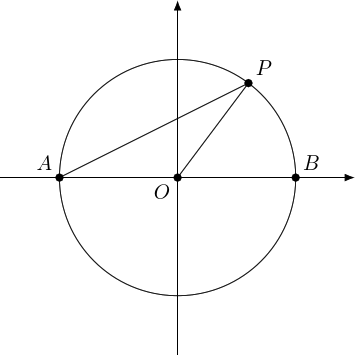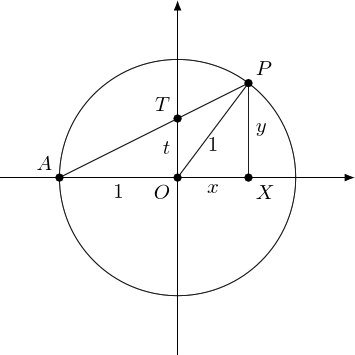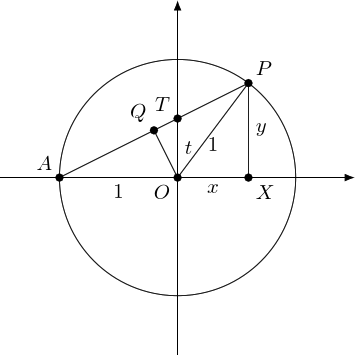Pythagorean Triangles

loopspace

1st October 2016

# 1 Introduction

Two things juxtaposed for this post. The first was learning from Niles Johnson of a way to generate Pythagorean triples using lines and circles. The second was learning that there are $320$ concepts to learn in order to take GCSE Mathematics.

One thing in the post about Pythagorean triples was the occurrence of the formulae:

 $x=\frac{1-{t}^{2}}{1+{t}^{2}},\phantom{\rule{2em}{0ex}}$

Now, it might just be the generation that I am, but I remember learning those formulae at school as how to write cosine and sine in terms of the tangent of the half angle. The formula book1 even made the substitution $t=\mathrm{tan}\left(x/2\right)$ so that the formulae read:

1I can still visualise the page all these years later.

 $\mathrm{cos}\left(x\right)=\frac{1-{t}^{2}}{1+{t}^{2}},\phantom{\rule{2em}{0ex}}$

So when reading that post, I wondered if there were some way to exploit that relationship to simplify the argument.

I had a go at it, and found what I believe is such a simplification.

In doing that, I realised that yet again similar triangles are the key. Which brings me to those $320$ concepts. How many of them, I wonder, are just "similar triangles" dressed up in strange language?

# 2 Pythagorean Triples

Let's go through the first part of the search for Pythagorean triples. A Pythagorean triple, let's recall, is a set of three integers, say $a$, $b$, and $c$, which satisfy Pythagoras' theorem:

 ${a}^{2}+{b}^{2}={c}^{2}.$

This means that there is a right-angled triangle with integer sides.

Dividing through by ${c}^{2}$ yields:

 $\frac{{a}^{2}}{{c}^{2}}+\frac{{b}^{2}}{{c}^{2}}=1$

and this means that $\left(\frac{a}{c},\frac{b}{c}\right)$ are the coordinates of a point on the unit circle with the property that both coordinates are rational numbers.

Conversely, if $\left(x,y\right)$ is a point on the unit circle with both $x$ and $y$ rational, then we can put them over a common denominator and so write $x=\frac{a}{c}$ and $y=\frac{b}{c}$, then as they are on the unit circle we have ${x}^{2}+{y}^{2}=1$ which untangles to ${a}^{2}+{b}^{2}={c}^{2}$.

So Pythagorean triples almost correspond to rational points on the unit circle. The "almost" is because if we multiply each number in a Pythagorean triple by the same constant then we get a new triple but they both give the same point on the unit circle. For example, $3,4,5$ and $6,8,10$ are different Pythagorean triples but $\left(3/5,4/5\right)$ and $\left(6/10,8/10\right)$ are the same point. We'll not bother about this.

In Niles Johnson's post, a diagram like Figure 1 appears.Figure 1: The Source of the Half Angle

In this, we can see the source of the half angle. The "angle at circumference is half angle at centre" rule leads to the conclusion that $P\stackrel{^}{A}O$ is half of $P\stackrel{^}{O}B$. So we could use some trigonometry to figure out the coordinates of $P$ in terms of the tangent of the angle at $P\stackrel{^}{A}O$, and once we had that we would have the formulae from Niles Johnson's post.

# 3 Similar Triangles

But trigonometry is just another name for similar triangles. So is there a way to use similar triangles directly?

The tangent of the angle at $P\stackrel{^}{A}O$ is the gradient of the line $AP$. Let's call this $t$. This is the height that the line rises for every one unit we go across. As we go across one unit from $A$ to $O$, the line $AP$ must cross the $y$–axis at height $t$. So we can also think of $t$ as the $y$–intercept of $AP$.

Our goal is the coordinates of $P$. It's a common thing to do to draw a right-angled triangle to illustrate the coordinates of a point, so let's adorn our diagram with that triangle. Adding both of these new features, we end up with Figure 2.Figure 2: The Labelled Diagram

There are two obvious similar triangles here: $ATO$ and $APX$. We could exploit this similarity together with the fact that $\left(x,y\right)$ lies on the circle to find the formulae for $x$ and $y$. Pythagoras tells us that ${x}^{2}+{y}^{2}=1$, while similarity says that $y=t\left(x+1\right)$. But then we need to substitute in and solve, which leads to a quadratic. This is Niles Johnson's approach.

We can do better.

There's another similar triangle. Let's "drop" the perpendicular from $O$ to $AP$. As triangle $AOP$ is isosceles (since $AO=1=OP$), this bisects $AP$. Figure 3 is the result.Figure 3: With the Perpendicular

Now $O\stackrel{^}{Q}A$ is a right-angle and so triangle $AQO$ is similar to $AOT$. What's a bit confusing here is that the triangles have been flipped, so the hypotenuse of $AQO$ is the base of $AOT$. Similarity then says:

 $\frac{AQ}{AO}=\frac{AO}{AT}.$

As $AQ$ bisects $AP$, and using $AO=1$, this means that:

 $AP=\frac{2}{AT}.$

Similarity also tells us that:

 $\frac{XP}{AP}=\frac{OT}{AT}.$

Substituting in $y=XP$ and $t=OT$, we get:

 $\frac{y}{AP}=\frac{t}{AT}.$

Putting these together yields:

 $y=t\frac{AP}{AT}=\frac{2t}{A{T}^{2}}.$

Finally, $AT$ is the hypotenuse of the right-angled triangle $AOT$ so $A{T}^{2}={1}^{2}+{t}^{2}$ and we get:

 $y=\frac{2t}{1+{t}^{2}}.$

And using similarity again tells us that $y=t\left(1+x\right)$ which leads to:

 $t\left(1+x\right)=\frac{2t}{1+{t}^{2}}$

and this rearranges to:

 $x=\frac{2}{1+{t}^{2}}-1=\frac{2-\left(1+{t}^{2}\right)}{1+{t}^{2}}=\frac{1-{t}^{2}}{1+{t}^{2}}.$

# 4 Rational Points

The purpose of this is that if $t\in ℚ$ then so also are $\frac{2t}{1+{t}^{2}}$ and $\frac{1-{t}^{2}}{1+{t}^{2}}$, meaning that the point $P$ has rational coordinates and we get a Pythagorean triple.

In fact, if we write $t=\frac{p}{q}$, then

 $x=\frac{1-\frac{{p}^{2}}{{q}^{2}}}{1+\frac{{p}^{2}}{{q}^{2}}}=\frac{{q}^{2}-{p}^{2}}{{q}^{2}+{p}^{2}},$

and

 $y=\frac{2\frac{p}{q}}{1+\frac{{p}^{2}}{{q}^{2}}}=\frac{2pq}{{q}^{2}+{p}^{2}}.$

This fits our pattern with $a={q}^{2}-{p}^{2}$, $b=2pq$, and $c={q}^{2}+{p}^{2}$, and thus this is our Pythagorean triple.

A bonus from this approach is that it shows that points in the unit circle with rational coordinates are dense. Pick a point $R$ on the circle with coordinates $\left(u,v\right)$. Then join $R$ to $A$. The slope of this line is $\frac{v}{1+u}$. Now choose $t$ to be a rational approximation to $\frac{v}{1+u}$. Then the resulting point $\left(x,y\right)$ is a rational approximation to $\left(u,v\right)$ lying on the unit circle.

And all from similar triangles!